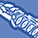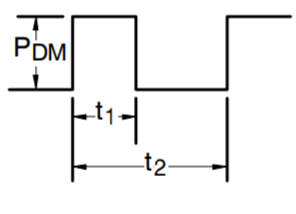cancel
Showing results for
Did you mean:

Power MOSFETNew Contributor II

IRF520N Switching Time Test Circuit

Dear Community,

I am trying to recreate the circuit shown below:

The data supplied from the Electrical Characteristics Table of the Infineon IRF520 MOSFET data sheet shows the following selection:

VDD = 50V
ID = 5.7A
RG = 22Ω
RD = 8.6Ω, See Fig. 10

I am using a signal generator which produces a pulse width of 1us and 10V peak.

However, I am not familiar with the concept of Duty Factor and that it has to be <= 0.1% can anyone explain that concept. What needs to be configured at the signal generator to achieve this duty factor?

I have looked at www.wikipedia.org and found the following explanation:

“The duty factor for periodic signal expresses the same notion, but is usually scaled to a maximum of one rather than 100%”

But according to the schematic, the Duty Factor is a percentage of 0.1%. Which is a contradiction.

1 SolutionModerator

Re: IRF520N Switching Time Test Circuit

Hi,

The duty factor / duty cycle expresses/determines how long the gate signal is turned ON in a given time.

duty cycle / duty factor is the fraction of one period in which a signal or system is active. Duty cycle is commonly expressed as a percentage or a ratio. A period is the time it takes for a signal to complete an on-and-of cycle

The duty factor is basically calculated by the following formula,

d = ton / T

where,   ton = Total ON time  / Pulse width

T = Total time period of the gate pulse = 1/fsw ( fsw = switching frequency)

Thus, a 60% duty cycle means the signal is On 60% of the time but OFF for 40% of the time.From the attached image, t1 corresponds to ton and t2 corresponds to total time T.

Creating pulses with low variable duty cycles can be achieved using the burst mode feature of a function generator.

1) Set up the pulse

2) Set up burst mode

3) Set the burst rate

4) Set the burst source to internal.

The detailed steps to achieve this can be found in the below link,

Please do let me know if you face any further issues while generating a PWM signal using signal generator.

Regards,

Abhilash P

3 RepliesModerator

Re: IRF520N Switching Time Test Circuit

Hi,

The duty factor / duty cycle expresses/determines how long the gate signal is turned ON in a given time.

duty cycle / duty factor is the fraction of one period in which a signal or system is active. Duty cycle is commonly expressed as a percentage or a ratio. A period is the time it takes for a signal to complete an on-and-of cycle

The duty factor is basically calculated by the following formula,

d = ton / T

where,   ton = Total ON time  / Pulse width

T = Total time period of the gate pulse = 1/fsw ( fsw = switching frequency)

Thus, a 60% duty cycle means the signal is On 60% of the time but OFF for 40% of the time.From the attached image, t1 corresponds to ton and t2 corresponds to total time T.

Creating pulses with low variable duty cycles can be achieved using the burst mode feature of a function generator.

1) Set up the pulse

2) Set up burst mode

3) Set the burst rate

4) Set the burst source to internal.

The detailed steps to achieve this can be found in the below link,

Please do let me know if you face any further issues while generating a PWM signal using signal generator.

Regards,

Abhilash PNew Contributor II

Re: IRF520N Switching Time Test Circuit

Dear Abhilash_P,

If I understand correctly the duty factor and the duty period are the same.

So duty factor = duty period = 0.1%

for the schematic circuit.

Best regardsModerator

Re: IRF520N Switching Time Test Circuit

Hi,